### C++ Program to implement Huffman Coding Compression Algorithm

Introduction
Huffman coding (also known as Huffman Encoding) is an algorithm for doing data compression, and it forms the basic idea behind file compression. This post talks about the fixed-length and variable-length encoding, uniquely decodable codes, prefix rules, and Huffman Tree construction.We already know that every character is sequences of 0's and 1's and stored using 8-bits this is known as fixed-length encoding as each character uses the same number of fixed-bit storage.

The technique works by creating a binary tree of nodes. A node can be either a leaf node or an internal node. Initially, all nodes are leaf nodes, which contain the character itself, the weight (frequency of appearance) of the character. Internal nodes contain character weight and links to two child nodes. As a common convention, bit `0` represents following the left child, and a bit `1` represents following the right child. A finished tree has `n` leaf nodes and `n-1` internal nodes. It is recommended that Huffman Tree should discard unused characters in the text to produce the most optimal code lengths.

We will use a priority queue for building Huffman Tree, where the node with the lowest frequency has the highest priority. Following are the complete steps:

1. Create a leaf node for each character and add them to the priority queue.

2. While there is more than one node in the queue:

Remove the two nodes of the highest priority (the lowest frequency) from the queue.

Create a new internal node with these two nodes as children and a frequency equal to the sum of both nodes’ frequencies.

Add the new node to the priority queue.

3. The remaining node is the root node and the tree is complete.

Consider some text consisting of only 'A', 'B', 'C', 'D', and 'E' characters, and their frequencies are  respectively. The following15, 7, 6, 6, 5, figures illustrate the steps followed by the algorithm: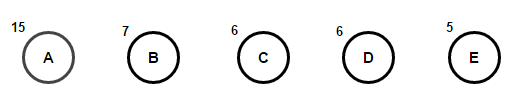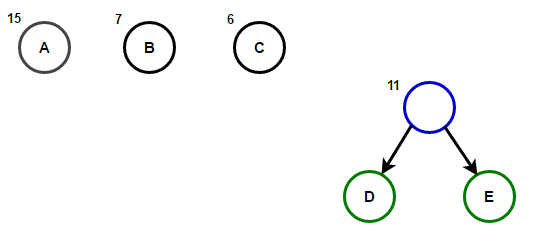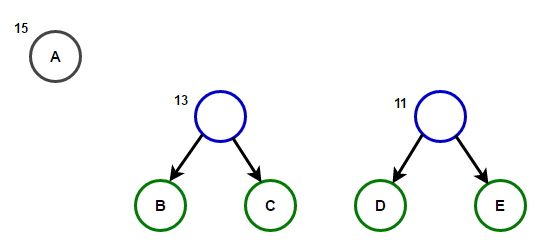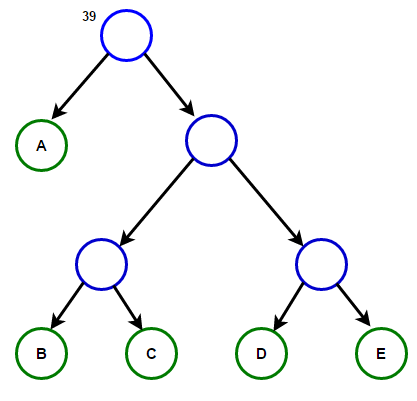The path from the root to any leaf node stores the optimal prefix code (also called Huffman code) corresponding to the character associated with that leaf node.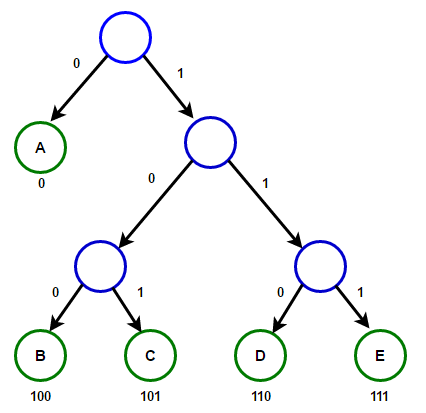Program:
#include <iostream>
#include <string>
#include <queue>
#include <unordered_map>
using namespace std;

#define EMPTY_STRING ""

// A Tree node
struct Node
{
char ch;
int freq;
Node *left, *right;
};

// Function to allocate a new tree node
Node* getNode(char ch, int freq, Node* left, Node* right)
{
Node* node = new Node();

node->ch = ch;
node->freq = freq;
node->left = left;
node->right = right;

return node;
}

// Comparison object to be used to order the heap
struct comp
{
bool operator()(const Node* l, const Node* r) const
{
// the highest priority item has the lowest frequency
return l->freq > r->freq;
}
};

// Utility function to check if Huffman Tree contains only a single node
bool isLeaf(Node* root) {
return root->left == nullptr && root->right == nullptr;
}

// Traverse the Huffman Tree and store Huffman Codes in a map.
void encode(Node* root, string str, unordered_map<char, string> &huffmanCode)
{
if (root == nullptr) {
return;
}

// found a leaf node
if (isLeaf(root)) {
huffmanCode[root->ch] = (str != EMPTY_STRING) ? str : "1";
}

encode(root->left, str + "0", huffmanCode);
encode(root->right, str + "1", huffmanCode);
}

// Traverse the Huffman Tree and decode the encoded string
void decode(Node* root, int &index, string str)
{
if (root == nullptr) {
return;
}

// found a leaf node
if (isLeaf(root))
{
cout << root->ch;
return;
}

index++;

if (str[index] == '0') {
decode(root->left, index, str);
}
else {
decode(root->right, index, str);
}
}

// Builds Huffman Tree and decodes the given input text
void buildHuffmanTree(string text)
{
// base case: empty string
if (text == EMPTY_STRING) {
return;
}

// count the frequency of appearance of each character
// and store it in a map
unordered_map<char, int> freq;
for (char ch: text) {
freq[ch]++;
}

// Create a priority queue to store live nodes of the Huffman tree
priority_queue<Node*, vector<Node*>, comp> pq;

// Create a leaf node for each character and add it
// to the priority queue.
for (auto pair: freq) {
pq.push(getNode(pair.first, pair.second, nullptr, nullptr));
}

// do till there is more than one node in the queue
while (pq.size() != 1)
{
// Remove the two nodes of the highest priority
// (the lowest frequency) from the queue

Node* left = pq.top(); pq.pop();
Node* right = pq.top();    pq.pop();

// create a new internal node with these two nodes as children and
// with a frequency equal to the sum of the two nodes' frequencies.
// Add the new node to the priority queue.

int sum = left->freq + right->freq;
pq.push(getNode('\0', sum, left, right));
}

// `root` stores pointer to the root of Huffman Tree
Node* root = pq.top();

// Traverse the Huffman Tree and store Huffman Codes
// in a map. Also, print them
unordered_map<char, string> huffmanCode;
encode(root, EMPTY_STRING, huffmanCode);

cout << "Huffman Codes are:\n" << endl;
for (auto pair: huffmanCode) {
cout << pair.first << " " << pair.second << endl;
}

cout << "\nThe original string is:\n" << text << endl;

// Print encoded string
string str;
for (char ch: text) {
str += huffmanCode[ch];
}

cout << "\nThe encoded string is:\n" << str << endl;
cout << "\nThe decoded string is:\n";

if (isLeaf(root))
{
// Special case: For input like a, aa, aaa, etc.
while (root->freq--) {
cout << root->ch;
}
}
else {
// Traverse the Huffman Tree again and this time,
// decode the encoded string
int index = -1;
while (index < (int)str.size() - 1) {
decode(root, index, str);
}
}
}

// Huffman coding algorithm implementation in C++
int main()
{
string text = "Huffman coding is a data compression algorithm.";
buildHuffmanTree(text);

return 0;
}

Output:

Huffman Codes are:

c 11111
h 111100
f 11101
r 11100
t 11011
p 110101
i 1100
g 0011
l 00101
a 010
o 000
d 10011
H 00100
. 111101
s 0110
m 0111
e 110100
101
n 1000
u 10010

The original string is:
Huffman coding is a data compression algorithm.

The encoded string is:
00100100101110111101011101010001011111100010011110010000011101110001101010101011001101011011010101111110000111110101111001101000110011011000001000101010001010011000111001100110111111000111111101

The decoded string is:
Huffman coding is a data compression algorithm#### More Articles of Shaik Aftab Ahmed:

Name Views Likes
C++ Program to Find the Frequency of Odd & Even Numbers in the given Matrix 390 1
C++ program to Sort a Linked List Using Merge Sort 358 1
C++ Program to Implement a Linked List representation of a Binary tree 350 1
C++ Program to Check for balanced parentheses in an expression 245 1
C++ Program to Perform Inorder, Preorder, Postorder traversals in a binary tree. 288 1
C++ program to print Indian flag 384 1
C++ program to Convert a multi level linked list to a singly linked list 265 1
C++ program to print right view of a Binary tree using queue 241 1
C++ Program to implement Huffman Coding Compression Algorithm 1625 1
C++ Program to Create a Height Balanced Binary Tree 274 1
C++ program to implement Prims algorithm 600 1
C++ Program for BFS Traversal 288 1
C++ Progam to Evaluate a Prefix Expression 450 1
C++ Program to Implement Queue using Linked List 250 1
C++ implementation of Stack using Linked list 299 1
C++ program to find the intersection point of two linked lists 331 1
C++ program to count the inversions in the given array 277 1
C++ program to perform D.N.F sort 325 1
C++ program to print all possible subsets of a String 286 1
C++ program to count the number of ones in a binary representation of a number 305 1
C++ program to print all possible subsets of a set 322 1
C++ program to find the largest word in a String 284 1
C++ Program to print a matrix in Spiral order 360 1
C++ program to convert from Binary to Decimal, Octal to Decimal, Hexadecimal to Decimal 311 1
C limits library 338 1
Program to add two Binary numbers 329 1